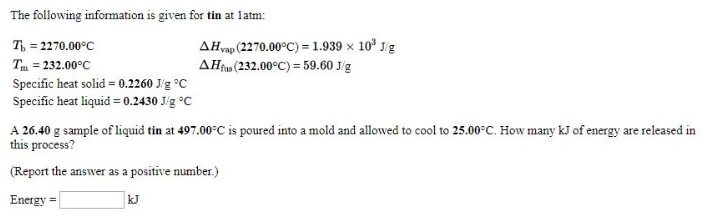# Problem: The following information is given for tin at 1 atm: Tb = 2270.00°C                      ΔHvap (2270.00°C) = 1.939 x 103 J/g Tm = 232.00°C                        ΔHfus (232.00°C) = 59.60 J/g Specific heat solid = 0.2260 J/g°C Specific heat liquid = 0.2430 J/g°C A 26.40 g sample of liquid tin at 497.00°C is poured into a mold and allowed to cool to 25.00°C. How many kJ of energy are released in this process? (Report the answer as a positive number.)

🤓 Based on our data, we think this question is relevant for Professor Block's class at Central Piedmont Community College.

###### FREE Expert Solution###### Problem Details

The following information is given for tin at 1 atm:

Tb = 2270.00°C                      ΔHvap (2270.00°C) = 1.939 x 103 J/g

Tm = 232.00°C                        ΔHfus (232.00°C) = 59.60 J/g

Specific heat solid = 0.2260 J/g°C

Specific heat liquid = 0.2430 J/g°C

A 26.40 g sample of liquid tin at 497.00°C is poured into a mold and allowed to cool to 25.00°C. How many kJ of energy are released in this process? (Report the answer as a positive number.)